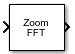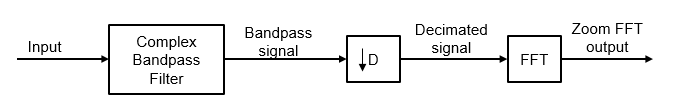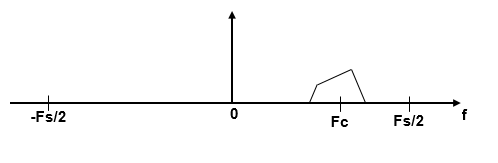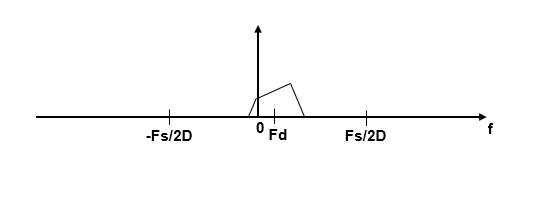Main Content

# Zoom FFT

High-resolution FFT of a portion of a spectrum

• Library:
• DSP System Toolbox / Transforms

•## Description

The Zoom FFT block computes the fast Fourier Transform (FFT) of a signal over a portion of frequencies in the Nyquist interval. By setting an appropriate decimation factor D, and sampling rate Fs, you can choose the bandwidth of frequencies to analyze BW, where BW = Fs/D. You can also select a specific range of frequencies to analyze in the Nyquist interval by choosing the center frequency of the desired band.

The resolution of a signal is the ratio of Fs and the FFT length (L). Using zoom FFT, you can retain the same resolution you would achieve with a full-size FFT on your original signal by computing a small FFT on a shorter signal. The shorter signal comes from decimating the original signal. The savings come from being able to compute a much shorter FFT while achieving the same resolution. For a decimation factor of D, the new sampling rate, Fsd, is Fs/D, and the new frame size (and FFT length) is Ld = L/D. The resolution of the decimated signal is Fsd/Ld = Fs/L. To achieve a higher resolution of the shorter band, use the original FFT length, L, instead of the decimated FFT length, Ld.

## Ports

### Input

expand all

Data input whose zoom FFT the block computes, specified as a vector or a matrix. The number of input rows must be a multiple of the decimation factor.

This block supports variable-size input signals, as long as the input frame size is a multiple of the decimation factor. That is, you can change the input frame size (number of rows) during the simulation. However, the number of channels (number of columns) must remain constant.

This port is unnamed until you select the Specify center frequency from input port parameter and click Apply.

Example: `randn(22,2)`

Data Types: `single` | `double`
Complex Number Support: Yes

Center frequency of the desired band in Hz, passed through this input port as a real scalar in the range (– SampleRate/2, SampleRate/2). SampleRate is the input sample rate either inherited from the input signal or specified through the Input sample rate (Hz) parameter. This port appears only when you select the Specify center frequency from input port check box.

This port appears only when you select the Specify center frequency from input port check box and click Apply.

Example: `0`

Example: `1200`

Data Types: `single` | `double`

### Output Arguments

expand all

Zoom FFT output, returned as a vector or matrix. If you select the Inherit FFT Length from input dimensions check box, the output frame size equals the input frame size divided by the decimation factor. If you clear the Inherit FFT Length from input dimensions check box and specify the FFT length, the output frame size equals the specified FFT length. The output data type matches the input data type.

Example: randn(11,2)

Data Types: `single` | `double`
Complex Number Support: Yes

## Parameters

expand all

Decimation factor, specified as a positive integer. This value specifies the factor by which the block reduces the bandwidth of the input signal. The number of rows in the input signal must be a multiple of the decimation factor.

Example: `4`

Example: `8`

Data Types: `single` | `double` | `int8` | `int16` | `int32` | `int64` | `uint8` | `uint16` | `uint32` | `uint64`

When you select this option and click , the input port Fc appears on the block icon. You can pass the center frequency through this input port as a scalar.

Center frequency of the desired band in Hz, specified as a real scalar in the range (– SampleRate/2, SampleRate/2). SampleRate is the input sample rate either inherited from the input or specified through the Input sample rate (Hz) parameter.

Example: `0.5`

Example: `10`

#### Dependencies

This parameter applies when you clear the Specify center frequency from input port check box.

Data Types: `single` | `double`

When you select this option, the FFT length is the ratio of the input frame size (number of rows in the input) and the Decimation factor.

FFT length, specified as a positive integer. The FFT length must be greater than or equal to the ratio of the frame size (number of input rows) and the Decimation factor.

Example: `24`

Example: `52`

#### Dependencies

This parameter applies when you clear the Inherit FFT Length from input dimensions check box.

Data Types: `single` | `double` | `int8` | `int16` | `int32` | `int64` | `uint8` | `uint16` | `uint32` | `uint64`

When you clear this check box, the block inherits the sample rate from the input signal.

Input sample rate in Hz, specified as positive real scalar.

Example: `44100`

Example: `48000`

• `Interpreted execution` — Simulate model using the MATLAB®  interpreter. This option shortens startup time and has faster simulation speed than ```Code generation```.

• `Code generation` — Simulate model using generated C code. The first time you run a simulation, Simulink® generates C code for the block. The C code is reused for subsequent simulations, as long as the model does not change. This option requires additional startup time and provides slower simulation speed than ```Interpreted execution```.

## Block Characteristics

 Data Types `double` | `single` Multidimensional Signals `No` Variable-Size Signals `Yes`

## Algorithms

The zoom FFT algorithm leverages bandpass filtering before computing the FFT of the signal. The concept of bandpass filtering is that suppose you are interested in the band [F1, F2] of the original input signal, sampled at the rate Fs Hz. If you pass this signal through a complex (one-sided) bandpass filter centered at Fc = (F1+F2)/2, with the bandwidth BW = F2F1, and then downsample the signal by a factor of D = floor(Fs/BW), the desired band comes down to the baseband.If Fc cannot be expressed in the form of k×Fs/D, where k is an integer, then the shifted, decimated spectrum is not centered at DC. In this case, the center frequency gets translated to Fd.

`${F}_{d}={F}_{c}-\left({F}_{s}/D\right)×floor\left(\left(D×{F}_{c}+{F}_{s}/2\right)/{F}_{s}\right)$`

The complex bandpass filter is obtained by first designing a lowpass filter prototype and then multiplying the lowpass coefficients with a complex exponential. This algorithm uses a multirate, multistage FIR filter as the lowpass filter prototype. To obtain the bandpass filter, the coefficients of each stage are frequency shifted. The decimation factor is the cumulative decimation factor of each stage. The complex bandpass filter followed by the decimator are implemented using an efficient polyphase structure. For more details on the design of the complex bandpass filter from the multirate multistage FIR filter prototype, see Zoom FFT and Complex Bandpass Filter Design.

 Harris, F.J. Multirate Signal Processing for Communication Systems. Prentice Hall, 2004, pp. 208–209.

Watch now